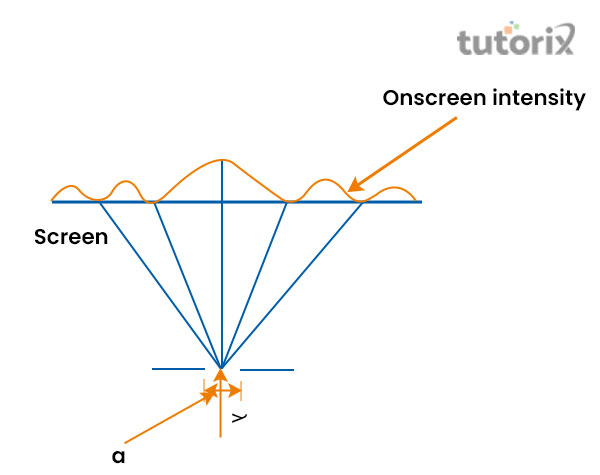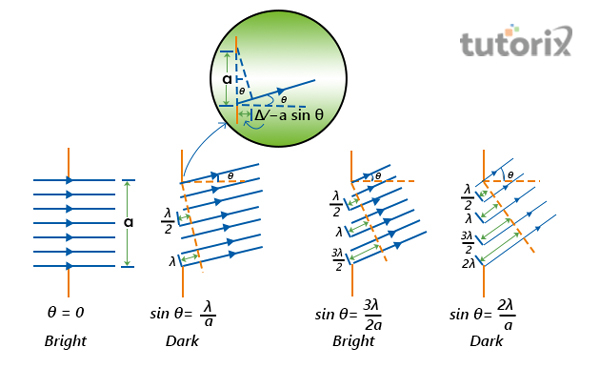# Single Slit Diffraction

## Introduction

The phenomenon of pressing waves around obstacles is commonly known by the term diffraction, based on this simple concept of physics, the present tutorial will explain the definition of a single-slit diffraction while explaining the pattern of diffraction. Furthermore, the tutorial will include an explanation of the formula of single-slit diffraction along with defining the central maximum as well. The calculation of single-slit diffraction will be included in the tutorial.

## What is single slit diffraction?Figure 1: Single-slit diffraction pattern

The bending phenomenon of light is generally observed in the experiment of Single-slit diffraction. The experiment proves that the light waves have a specific wavelength and the frequency of these wavelengths can be changed when a reaction happens in the wave of light while travelling through a small slit (Qi & Wong, 2022).

This change observed in the frequency often produces a distinctive pattern on the screen placed behind the slit that is called the diffracted pattern.

## Single slit diffraction: Formula

The formula of the single-slit diffraction can be represented through mathematical representation. For better understanding, it is conjectured that the width of the slit is

The angular position of any point on the screen that is $\mathrm{\vartheta}$ has the measurement that is found by the centre of slit that divides the slit by the length of a/2 (Animations.physics.unsw, 2022). Considering that, the emanation of two rays takes place at a distance of a/2 from each other. Based on this, the formula that can be expressed mathematically is

ΔL = a/2sinθ.

For the dark fringe, the wavelength is calculated between λ2 and λ. Therefore, the formula of the first fringe will be ΔL = λ2 = a/2sinθ where λ = a sin θ and for the second minima, the formula will be λ/2 = a/4sin θ where 2λ = a sin θ.

## The Central Maximum

`The position of maxima is identified in between the width and the minima of the central context (Yin, He & Wu, 2020). Based on this concept it can be explained in a simple diction that the central maximum can be referred to as the distance that lies between first-order minima from the central position of the screen that is existed on both the sides of the centre.

In such cases, the position of the minima is generally expressed by the y, and the measurement the width of which is generally measured from the centre of the screen. This leads to the development of the formula that is tanθ $\approx$ θ$\approx$ y/D. Based on this, the formula for small ϑ will be sin θ$\approx$θ. Following this i λ = a sin θ$\approx$aθ can be considered that leads to θ = y/D = λa or y = λDa. Now focusing on this to find the width of the central maximum, it will be considered that the width will be represented as 2λDa and the angular width of central maximum will be 2θ = 2λa.

## Single slit diffraction: calculationFigure 2: Analysing a graph of the single-slit diffraction pattern

It has been seen in the figure that the slit is narrow. This situation is considered a constant with the fact that the interaction of light must happen with an object that is comparable in size to its wavelength. It helps to exhibit the effect of waves of light such as the pattern of single-slit diffraction. The expansion of the central maximum is 20.7° on either side of the central place of the original beam as the width is 41°.

## Diffraction through a Single SlitFigure 3: Diffraction through a Single Slit

The above figure represents the experiment of Huygens’s principle that showcased that if the light can be focused on a single slit from different directions, the diffraction will be different or the different angular wavelength of the wave of light (Phys.libretexts, 2022). In this experiment, it has been seen that most of the rays after travelling through the slit have another ray to interfere in a constructive manner which is responsible for the occurrence of a maximum in intensity at this angle.

## Conclusion

Based on the concept of diffraction of waves, the tutorial has explained that a single-slit diffraction of light can be observed when light travels through a single slit. This has a width that is equivalent to the wavelength of light. In such cases, it has been observed that the maxima generally lie between the width and the minima of the central context.

Furthermore, the tutorial has represented the formula of the single-slit diffraction that is represented as a mathematical representation,ΔL = a/2sinθ.

## FAQs

Q1. What is meant by the diffraction minima and maxima?

Ans: Surrounded by bright and dark lines, the diffraction maxima is referred to as a central bright fringe that is generally known by the term central maxima. The surrounded dark and bright lines are referred to as the secondary minima.

Q2. Why does light diffract in a single slit?

Ans: At the time when the waves of light pass through a small slit, the width of which is similar to the wavelength, the part of the light that goes through the slit acts as a point source that is responsible for the generation of a spherical wave. This is the main reason why the light diffracts.

Q3. What is the wavelength of light?

Ans: When a wave of light passes through a specific phase, the crests are evolved in the path of the wave while travelling. The distance between these two crests is generally known by the term wavelength.

Q4. What is the core difference lies between a single-slit and a double-slit diffraction?

Ans: In the case of a single-slit diffraction, it has been observed that the maxima are smaller than the central maximum whereas, while passing through a double-slit, it produces evenly space lines that dims slowly on either side of the centre.